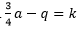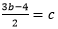#### MAT-HS.A-CED.04

 MAT-HS Targeted Standards(A) Concept: Algebra(CED) Domain: Creating ExpressionsCluster: Create equations that describe numbers or relationships MAT-HS.A-CED.04 Rearrange formulas to highlight a quantity of interest, using the same reasoning as in solving equations. For example, rearrange Ohm’s law V = IR to highlight resistance R.

## Student Learning Targets:

• I can
• I can

• I can
• I can

### Skills (Performance) Targets

• I can solve for (isolate) a specific variable.
• I can perform transformations that produce equivalent equations (e.g., adding the same amount to both sides of the equation, etc.)
• I can solve multi-variable formulas or literal equations for a specific variable.

• I can
• I can

## Proficiency Scale

 Score Description Sample Activity 4.0 (advanced) In addition to Score 3.0, the student demonstrates in-depth inferences and applications regarding more complex material that go beyond end of instruction expectations. Solve for h:   3h+g=5h-hg 3.5 In addition to Score 3.0 performance, the student demonstrates in-depth inferences and applications regarding the more complex content with partial success. 3.0 (proficient) The student can: solve multi-variable formulas or literal equations for a specific variable. The student exhibits no major errors or omissions. Solve the following equation for b: A = 1/2h (b+c) Solve the following equation for a:Solve the following equation for b:2.5 The student demonstrates no major errors or omissions regarding the simpler details and processes (Score 2.0 content) and partial knowledge of the more complex ideas and processes (Score 3.0 content). 2.0 (progressing) There are no major errors or omissions regarding the simpler details and processes as the student can: identify appropriate inverse operations. solve a simple linear equation for y. However, the student exhibits major errors or omissions regarding the more complex ideas and processes. Solve the following equation for y: 2x - 3y = 8 1.5 The student demonstrates partial knowledge of the simpler details and processes (Score 2.0 content) but exhibits major errors or omissions regarding the more complex ideas and procedures (Score 3.0 content). 1.0 (beginning) With help, the student demonstrates a partial understanding of some of the simpler details and processes (Score 2.0 content) and some of the more complex ideas and processes (Score 3.0 content). 0.5 With help, the student demonstrates a partial understanding of some of the simpler details and processes (Score 2.0 content) but not the more complex ideas and processes (Score 3.0 content). 0.0 Even with help, the student demonstrates no understanding or skill.

## Resources

 Web Vocab literal equation